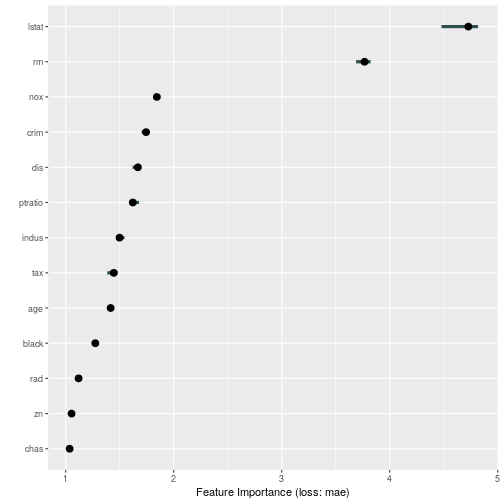The `iml` package can now handle bigger datasets. Earlier problems with exploding memory have been fixed for `FeatureEffect`, `FeatureImp` and `Interaction`. It's also possible now to compute `FeatureImp` and `Interaction` in parallel. This document describes how.

First we load some data, fit a random forest and create a Predictor object.

``````set.seed(42)
library("iml")
library("randomForest")
data("Boston", package = "MASS")
rf <- randomForest(medv ~ ., data = Boston, n.trees = 10)
X <- Boston[which(names(Boston) != "medv")]
predictor <- Predictor\$new(rf, data = X, y = Boston\$medv)
``````

## Going parallel

Parallelization is supported via the {future} package. All you need to do is to choose a parallel backend via `future::plan()`.

``````library("future")
library("future.callr")
# Creates a PSOCK cluster with 2 cores
plan("callr", workers = 2)
``````

Now we can easily compute feature importance in parallel. This means that the computation per feature is distributed among the 2 cores I specified earlier.

``````imp <- FeatureImp\$new(predictor, loss = "mae")
library("ggplot2")
plot(imp)
``````That wasn't very impressive, let's actually see how much speed up we get by parallelization.

``````bench::system_time({
plan(sequential)
FeatureImp\$new(predictor, loss = "mae")
})
#> process    real
#>   1.56s    1.3s
bench::system_time({
plan("callr", workers = 2)
FeatureImp\$new(predictor, loss = "mae")
})
#> process    real
#>   1.72s   3.92s
``````

A little bit of improvement, but not too impressive. Parallelization is more useful in the case where the model uses a lot of features or where the feature importance computation is repeated more often to get more stable results.

``````bench::system_time({
plan(sequential)
FeatureImp\$new(predictor, loss = "mae", n.repetitions = 20)
})
#> process    real
#>    5.1s   4.72s

bench::system_time({
plan("callr", workers = 2)
FeatureImp\$new(predictor, loss = "mae", n.repetitions = 20)
})
#> process    real
#>   1.72s   5.51s
``````

### Interaction

Here the parallel computation is twice as fast as the sequential computation of the feature importance.

The parallelization also speeds up the computation of the interaction statistics:

``````bench::system_time({
plan(sequential)
Interaction\$new(predictor)
})
#> process    real
#>   8.26s   7.87s
bench::system_time({
plan("callr", workers = 2)
Interaction\$new(predictor)
})
#> process    real
#>   1.72s   7.61s
``````

### Feature Effects

Same for `FeatureEffects`:

``````bench::system_time({
plan(sequential)
FeatureEffects\$new(predictor)
})
#> process    real
#>   945ms   823ms
bench::system_time({
plan("callr", workers = 2)
FeatureEffects\$new(predictor)
})
#> process    real
#>   6.88s   10.3s
``````